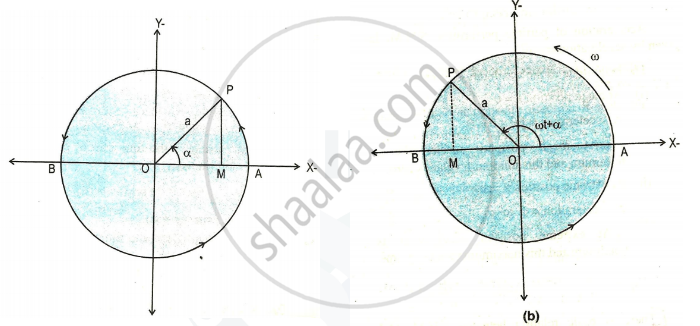# Show that S.H.M. is a Projection of U.C.M. on Any Diameter - Physics

Show that S.H.M. is a projection of  U.C.M. on any diameter

#### Solution

Linear S.H.M. is defined as the linear periodic motion of a body, in which the restoring force (or acceleration) is always directed towards the mean position and its magnitude is directly proportional to the displacement from the mean position.
There is basic relation between S.H.M. and U.C.M. that is very useful in understanding S.H.M. For an object performing U.C.M. the projection of its motion along any diameter of its path executes S.H.M.
Consider particle ‘P’ is moving along the circumference of circle of radius ‘a’ with constant angular speed of ω in anticlockwise direction as shown in figure.
Particle P along circumference of circle has its projection particle on diameter AB at point M. Particle P is called reference particle and the circle on which it moves, its  projection moves back and forth along the horizontal diameter, AB.The x–component of the displacement of P is always same as displacement of M, the x–component of the velocity of P is always same as velocity of M and the x–component of the acceleration of M.
Suppose that particle P starts from initial position with initial phase α (angle between radius OP and the x – axis at the time t = 0) In time t the angle between OP and x - axis is ( ωt + α ) as particle P moving with constant angular velocity (ω) as shown in figure.
cos( ωt + α ) = x/a

therefore x=acos(omegat+alpha)                       ......(1)

This is the expression for displacement of particle M at time t.
As velocity of the particle is the time rate of change of displacement then we have

v=dx/dt=d/dt[acos(omegat+alpha)]

therefore v=-aomegasin(omegat+alpha)            ......(2)

As acceleration of particle is the time rate of change of velocity, we have

a=(dv)/dt=d/dt [-aomegasin(omegat+alpha)]

thereforea=-aomega^2cos(omegat+alpha)

therefore a= omega^2x

It shows that acceleration of particle M is directly proportional to its displacement and its direction is opposite to that of displament. Thus particle M performs simple harmonic motion but M is projection of particle performing U.C.M. hence S.H.M. is projection of U.C.M. along a diameter, of circle.

Concept: Linear Simple Harmonic Motion (S.H.M.)
Is there an error in this question or solution?
Chapter 5: Oscillations - Exercises [Page 129]

#### APPEARS IN

Balbharati Physics 12th Standard HSC Maharashtra State Board
Chapter 5 Oscillations
Exercises | Q 4 | Page 129
Share